Stealth Silencer Plans This is a "competition silencer" because there is no obstruction to the free flow of gases through the silencer. It is a variation of an even better design from my friend in Russia who is trying to sell the design. But this one works very well. The 7/16" holes are for a 69cc engine. They should be 5/16" for the 48cc.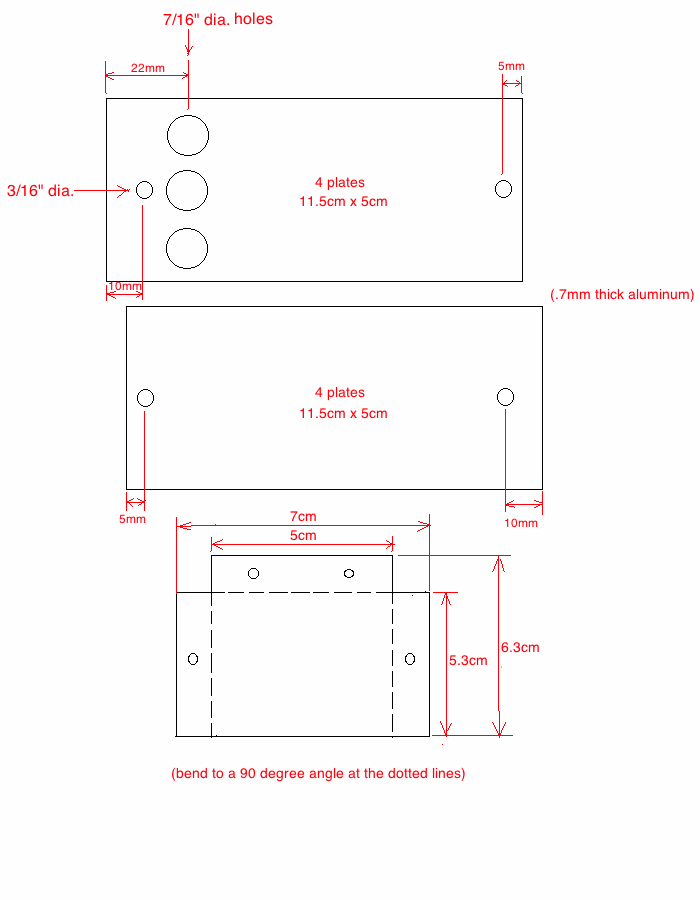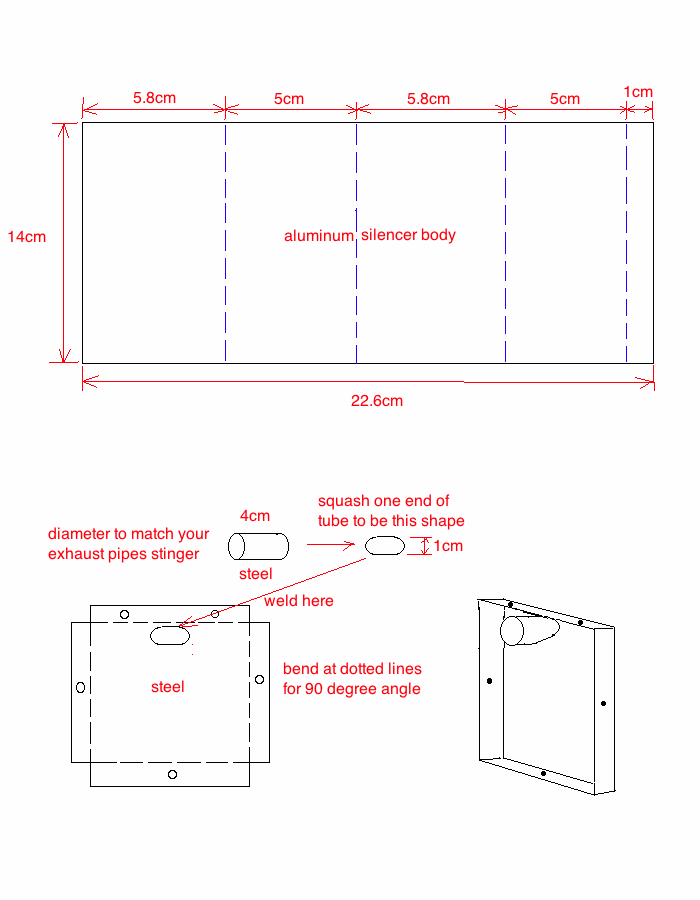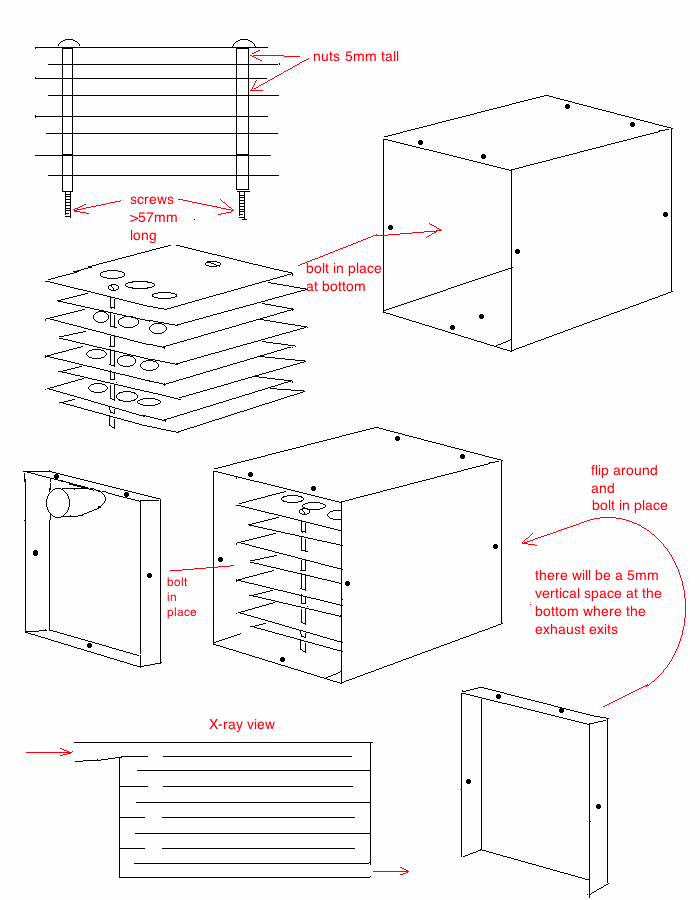Here is my last one being put together with aluminum plates and steel body: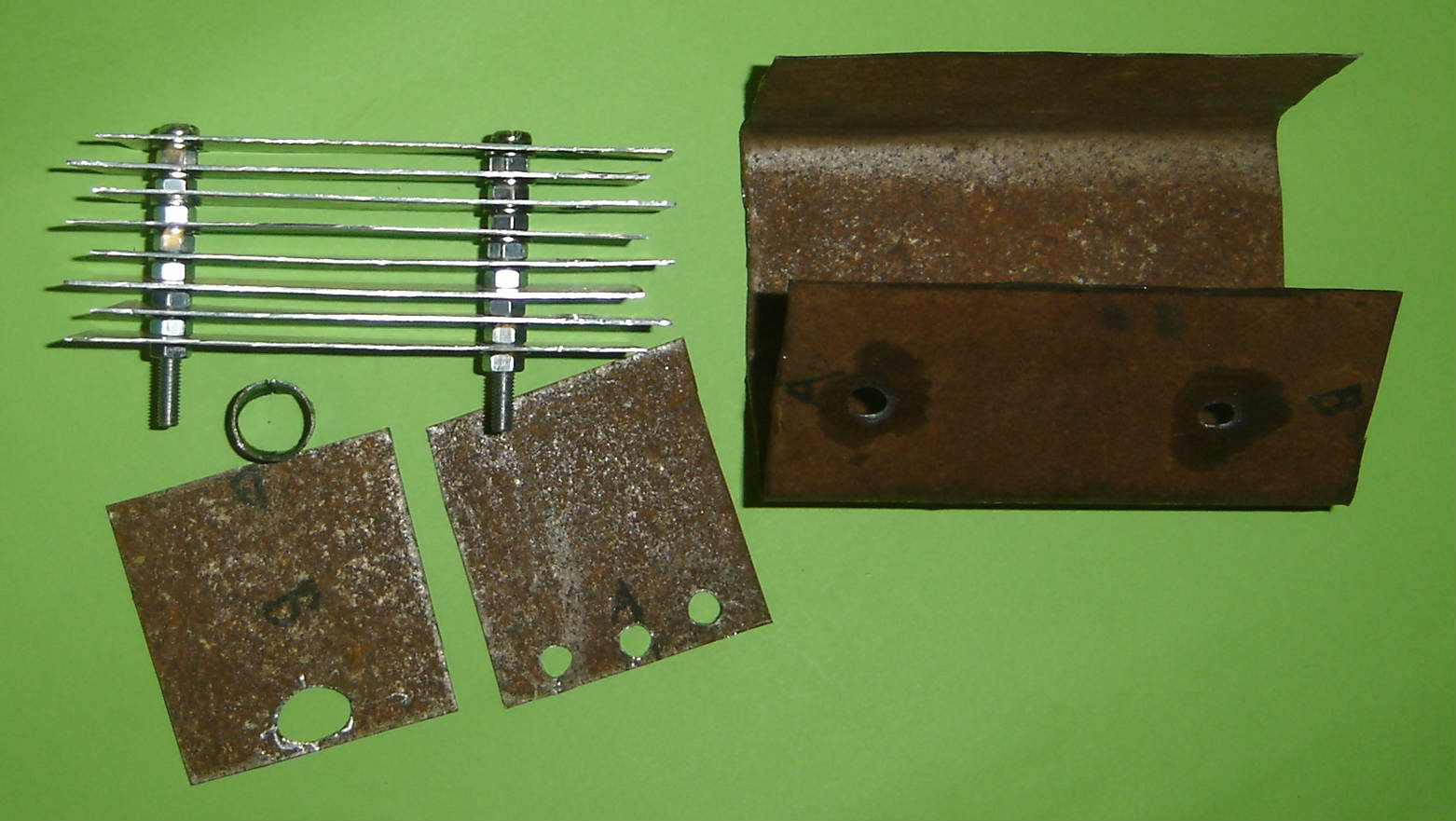Here is the finished product. I made two and am selling one of them. Here is its picture. Make an offer.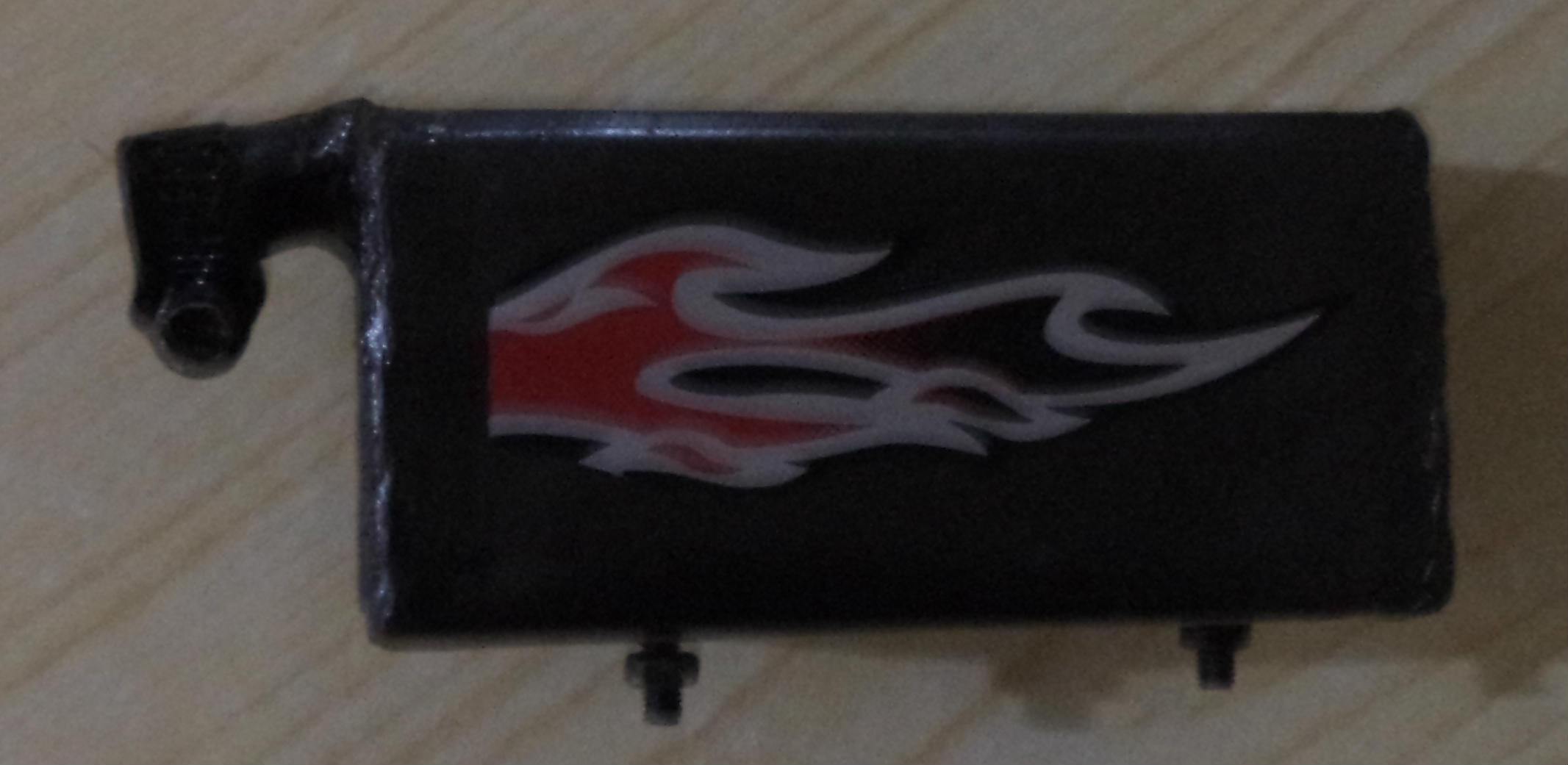TEST RESULTS WITH DECIBEL METER Decibels express a power ratio, not an amount. They tell how many times more (positive dB) or less (negative dB) but not how much in absolute terms. Decibels are logarithmic, not linear. For example, 20 dB is not twice the power ratio of 10 dB. A 10 db increase is 10 times the lower amount. The defining equation for decibels is db = 10*log10(P2/P1) where P1 is the power being measured, and P1 is the reference to which P2 is being compared. P2/P1 is the power ratio. The following measurements were at a 45 degree angle from the exiting exhaust pulses and 21 inches from the silencer. The engine was running at a fast idle. A normal expansion chamber with fairly restrictive silencer gave 94db which is a 2.5x10^9 power ratio*. My torque pipe + my stealth silencer gave 91db which is a 1.26x10^9 power ratio. The Power Ratio of 91db = 1/2 the Power Ratio of 94db so essentially my setup outputs half as much noise. * site for calculating power ratio from db is at http://www.mogami.com/e/cad/db.html HOME